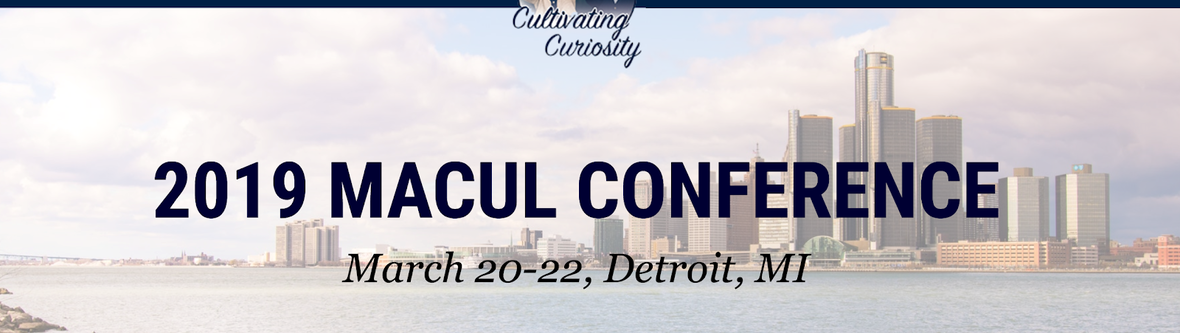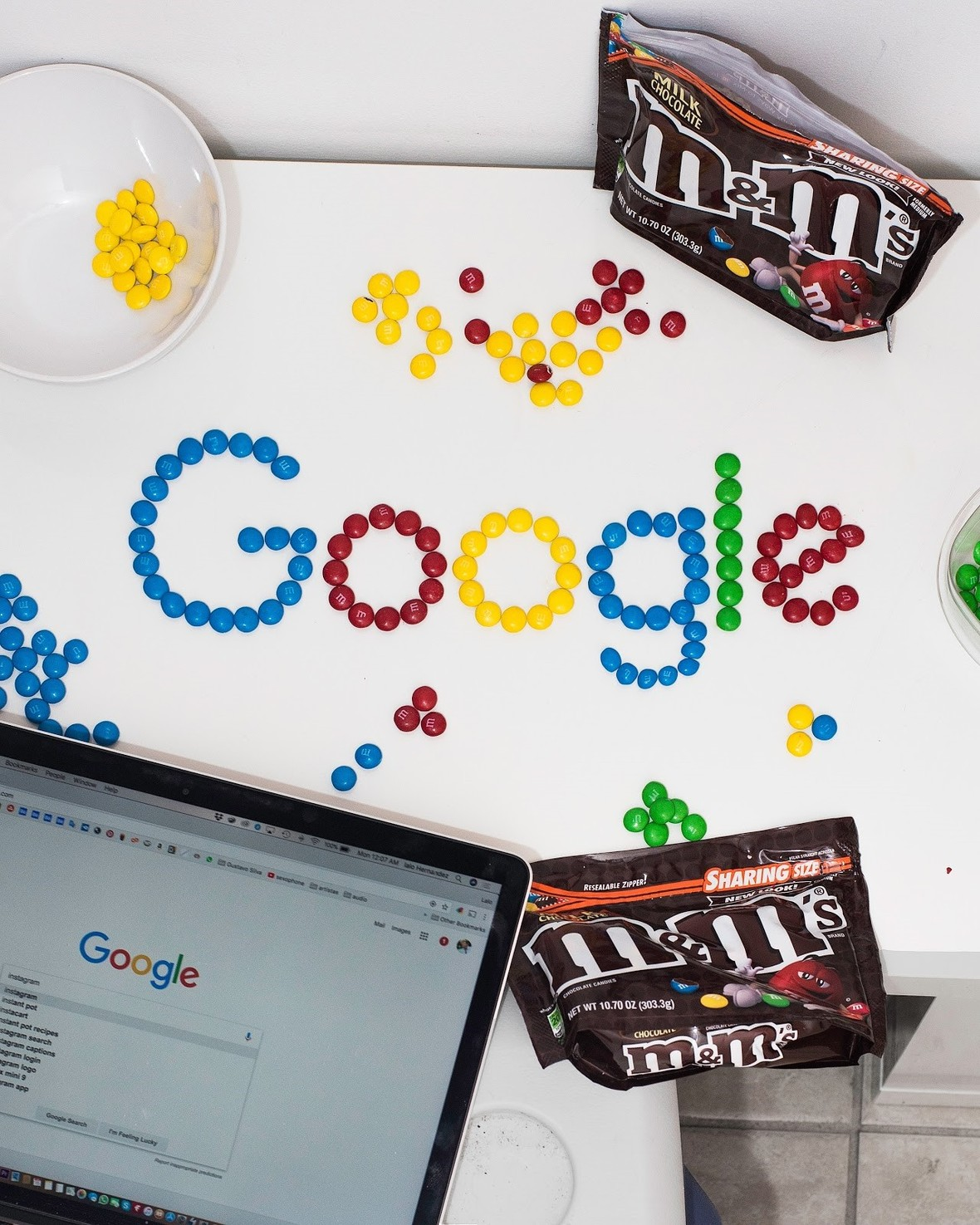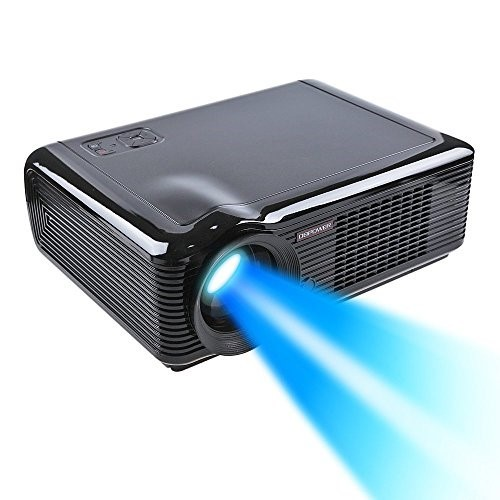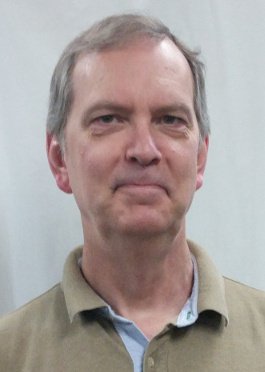Like   Tweet   +1
 /* styles */

## December 2018

 table div table+table+table+table div table{width:100%;padding:0}table div table+table+table+table div table img{width:96.23%;padding:0;float:none}table div table+table+table+table div table td{width:100%;padding:0 1.88% 18px}/* styles */## Classroom Maker ResourcesREMC1’s classroom maker kit has grown! We have added more Keva Planks, Ozobots, Bloxels, Spheros and a set of Makedos. To see what all is available and to reserve items for check out, please visit the Resource Request Form on our website at www.remc1.org/links.

 table div table+table+table+table+table+table+table div table{width:100%;padding:0}table div table+table+table+table+table+table+table div table img{width:96.23%;padding:0;float:none}table div table+table+table+table+table+table+table div table td{width:100%;padding:0 1.88% 18px}/* styles */## 2019 MACUL ConferenceThe MACUL Conference, Michigan's premier educational technology event, will be held March 20-22 at the Cobo Center in Detroit, MI. Over 5,000 educators attend this conference annually and they are anticipating another record year!

REMC1 recognizes the value of this conference and would like to assist our local schools by organizing a group discount registration and travel accommodations via a charter bus. We will also pay the registration fee and bus cost for the Tech Academy Coaches!

In order to receive the group discounted registration, REMC1 will need to register you with MACUL (DO NOT REGISTER ON MACUL's WEBSITE). Instead, please see our MACUL Registration & Bus Form. However, you will still need to book your hotel accommodations (nights of 3/20 & 3/21) on your own and please visit MACUL's Conference Hotel Web page for reservations.

Please let us know if you would like to attend by February 15 so we can make appropriate plans. Requests will be considered final at that point for billing purposes. The estimated cost for the bus ride is anticipated to be less than \$100 each but will be based on the total number of riders.

Additional information on the conference can be viewed at https://maculconference.org/ and questions can be directed to Steve Kass at steve@remc1.org.

 table div table+table+table+table+table+table+table+table+table+table div table{width:100%;padding:0}table div table+table+table+table+table+table+table+table+table+table div table img{width:96.23%;padding:0;float:none}table div table+table+table+table+table+table+table+table+table+table div table td{width:100%;padding:0 1.88% 18px}/* styles */## Upcoming WorkshopsREMC1 is currently working on our winter professional development offerings. We are anticipating offering a couple of Google related workshops in the December/January timeframe. Please stay tuned for the session topics and dates which will be posted to http://www.remc1.org/professional-development

 table div table+table+table+table+table+table+table+table+table+table+table+table+table div table{width:100%;padding:0}table div table+table+table+table+table+table+table+table+table+table+table+table+table div table img{width:96.23%;padding:0;float:none}table div table+table+table+table+table+table+table+table+table+table+table+table+table div table td{width:100%;padding:0 1.88% 18px}/* styles */## Giveaway Drawing!REMC1 has several older data projectors that are no longer needed. These projectors are VGA only (no digital or HDMI inputs). They are currently working, but provided 'as is', and do not come with any support.

If you are interested in acquiring one for school use, please fill out this projector giveaway form before December 16. We will draw random winners and notify them directly.

 table div table+table+table+table+table+table+table+table+table+table+table+table+table+table+table+table div table{width:100%;padding:0}table div table+table+table+table+table+table+table+table+table+table+table+table+table+table+table+table div table img{width:96.23%;padding:0;float:none}table div table+table+table+table+table+table+table+table+table+table+table+table+table+table+table+table div table td{width:100%;padding:0 1.88% 18px}/* styles */## Staff Spotlight!This month we are spotlighting Lauren Keller who began working at REMC1 in May. Lauren spent his youth surrounded by technology while growing up in Silicon Valley. He helped his friends program TRS-80 and Atari 800 computers in BASIC, as well as some QS Forth and Assembly. Though he had a knack for such things, he joined the Navy instead and moved to San Diego, where he would meet his future wife. This introduced Lauren to the lost continent of The Upper Peninsula, and it was here the technology field finally came into focus. Graduating in 2013 from Michigan Tech with a BS in Computer Network and System Administration, he spent 5 years at Michigan Tech before accepting a position at REMC1. Welcome Lauren!

 table div table+table+table+table+table+table+table+table+table+table+table+table+table+table+table+table+table+table+table div table{width:100%;padding:0}table div table+table+table+table+table+table+table+table+table+table+table+table+table+table+table+table+table+table+table div table img{width:96.23%;padding:0;float:none}table div table+table+table+table+table+table+table+table+table+table+table+table+table+table+table+table+table+table+table div table td{width:100%;padding:0 1.88% 18px}/* styles */## Teacher's CornerThe REMC Virtual Course registration is open. People can register for January - March courses. Here are the January courses:

▪ OER to Support Early Literacy
▪ Student-Designed OER for 21st Century Learning
▪ Inquiry and Questioning: Igniting Student Curiosity with Technology, Level 1
▪ Authentic Student Learning and Gamification
▪ Applying Google Apps for Education in Chrome, Level 1
▪ Transforming Education - Maker Movement
▪ Increasing Efficiency and Productivity
 ▪ OER to Support Early Literacy
 ▪ Student-Designed OER for 21st Century Learning
 ▪ Inquiry and Questioning: Igniting Student Curiosity with Technology, Level 1
 ▪ Authentic Student Learning and Gamification
 ▪ Applying Google Apps for Education in Chrome, Level 1
 ▪ Transforming Education - Maker Movement
 ▪ Increasing Efficiency and Productivity

The courses are free and open to Michigan school personnel. You can take courses “just to learn” or complete the course and assignments and earn SCECH credits. All are facilitated by Michigan educators. Each lasts three weeks and consists of two one-hour webinars and four to eight hours of resource review, assessment development, discussion boards and written reflection. SCECHs range from 6 - 10 depending on the course.

 table div table+table+table+table+table+table+table+table+table+table+table+table+table+table+table+table+table+table+table+table+table+table div table{width:100%;padding:0}table div table+table+table+table+table+table+table+table+table+table+table+table+table+table+table+table+table+table+table+table+table+table div table img{width:96.23%;padding:0;float:none}table div table+table+table+table+table+table+table+table+table+table+table+table+table+table+table+table+table+table+table+table+table+table div table td{width:100%;padding:0 1.88% 18px}/* styles */## ResourcesEduPaths is a free resource from TRIG created to centralize PD around the state. Find resources and get FREE SCECH hours!

Current REMC Connect (REMC Association of Michigan Newsletter)

Check out Discovery Streaming updates and enhancing instruction with streaming resources.

MeL is a great place for Michigan Teachers to find classroom resources. They have just recently launched MeL Teachers which offers eight centers that will help with site navigation and make important information easier to access.

REMC Virtual Courses, October, 2018. Free to Michigan school personnel. Sessions include: Open Education Resources, Google Apps for Chrome, Literacy and Technology, Social Media and more. SCECHs available. FAQs about the free REMC Virtual Courses

eSchool News-Teaching and Learning

 table div table+table+table+table+table+table+table+table+table+table+table+table+table+table+table+table+table+table+table+table+table+table+table+table+table div table{width:100%;padding:0}table div table+table+table+table+table+table+table+table+table+table+table+table+table+table+table+table+table+table+table+table+table+table+table+table+table div table img{width:96.23%;padding:0;float:none}table div table+table+table+table+table+table+table+table+table+table+table+table+table+table+table+table+table+table+table+table+table+table+table+table+table div table td{width:100%;padding:0 1.88% 18px}/* styles */## Connection Corner▪ Discovery Education upcoming events
▪ Video Conference field trips and Classroom collaborations
▪ iNACOL webinars
▪ ISTE blog and magazine
▪ EdTech magazine
▪ REMC SAVE
▪ Michigan Ed Tech - METS
 ▪ Discovery Education upcoming events
 ▪ Video Conference field trips and Classroom collaborations
 ▪ ISTE blog and magazine
 ▪ EdTech magazine
 ▪ REMC SAVE
 ▪ Michigan Ed Tech - METS
 table div table+table+table+table+table+table+table+table+table+table+table+table+table+table+table+table+table+table+table+table+table+table+table+table+table+table+table+table div table{width:100%;padding:0}table div table+table+table+table+table+table+table+table+table+table+table+table+table+table+table+table+table+table+table+table+table+table+table+table+table+table+table+table div table img{width:96.23%;padding:0;float:none}table div table+table+table+table+table+table+table+table+table+table+table+table+table+table+table+table+table+table+table+table+table+table+table+table+table+table+table+table div table td{width:100%;padding:0 1.88% 18px}/* styles */table div table+table+table+table+table+table+table+table+table+table+table+table+table+table+table+table+table+table+table+table+table+table+table+table+table+table+table+table+table+table div table{width:100%;padding:0}table div table+table+table+table+table+table+table+table+table+table+table+table+table+table+table+table+table+table+table+table+table+table+table+table+table+table+table+table+table+table div table img{width:96.23%;padding:0;float:none}table div table+table+table+table+table+table+table+table+table+table+table+table+table+table+table+table+table+table+table+table+table+table+table+table+table+table+table+table+table+table div table td{width:100%;padding:0 1.88% 18px}/* styles *//* styles */ The REMC office will be closed the following days during the holiday season: Monday, December 24, 2018 Tuesday, December 25, 2018 Monday, December 31, 2018 Tuesday, January 1, 2019
 table div table+table+table+table+table+table+table+table+table+table+table+table+table+table+table+table+table+table+table+table+table+table+table+table+table+table+table+table+table+table+table+table div table{width:100%;padding:0}table div table+table+table+table+table+table+table+table+table+table+table+table+table+table+table+table+table+table+table+table+table+table+table+table+table+table+table+table+table+table+table+table div table img{width:96.23%;padding:0;float:none}table div table+table+table+table+table+table+table+table+table+table+table+table+table+table+table+table+table+table+table+table+table+table+table+table+table+table+table+table+table+table+table+table div table td{width:100%;padding:0 1.88% 18px}/* styles */table div table+table+table+table+table+table+table+table+table+table+table+table+table+table+table+table+table+table+table+table+table+table+table+table+table+table+table+table+table+table+table+table+table+table div table{width:100%;padding:0}table div table+table+table+table+table+table+table+table+table+table+table+table+table+table+table+table+table+table+table+table+table+table+table+table+table+table+table+table+table+table+table+table+table+table div table img{width:96.23%;padding:0;float:none}table div table+table+table+table+table+table+table+table+table+table+table+table+table+table+table+table+table+table+table+table+table+table+table+table+table+table+table+table+table+table+table+table+table+table div table td{width:100%;padding:0 1.88% 18px}/* styles */table div table+table+table+table+table+table+table+table+table+table+table+table+table+table+table+table+table+table+table+table+table+table+table+table+table+table+table+table+table+table+table+table+table+table+table+table div table{width:100%;padding:0}table div table+table+table+table+table+table+table+table+table+table+table+table+table+table+table+table+table+table+table+table+table+table+table+table+table+table+table+table+table+table+table+table+table+table+table+table div table img{width:96.23%;padding:0;float:none}table div table+table+table+table+table+table+table+table+table+table+table+table+table+table+table+table+table+table+table+table+table+table+table+table+table+table+table+table+table+table+table+table+table+table+table+table div table td{width:100%;padding:0 1.88% 18px}/* styles */